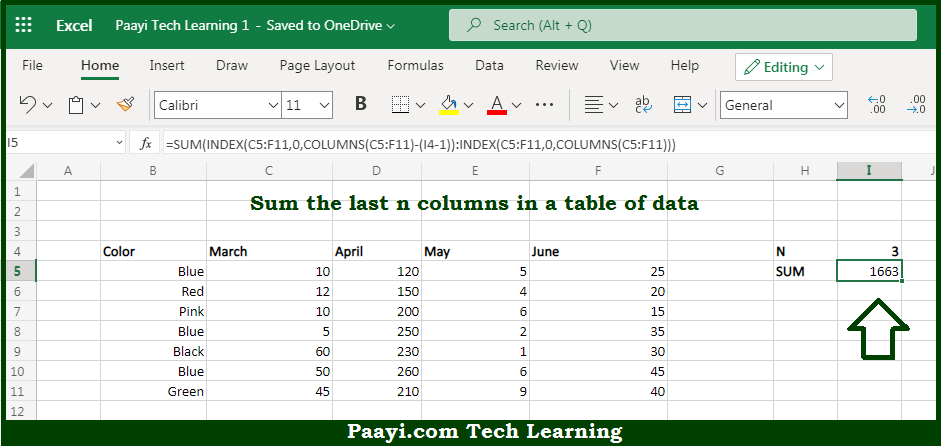# Learn How to SUM Last n Columns in Microsoft Excel

Written by | 0 Comments | 643 Views

In this article, you will learn how to SUM various things in Microsoft Excel using a single/combination(s) of functions. You will also know how to SUM Last n Columns and see the generic formula.

SUM Last n Columns in Microsoft Excel

The main purpose of this formula is to sum the last n columns in a table of data that implies the last 3 columns, or last 5 columns, etc. Here we will learn how to sum the last n columns in the workbook in Microsoft Excel. That implies, with the help of a formula based on the INDEX function you can able sum the last n columns in a table of data (i.e. last 3 columns, last 4 columns, etc. So, with the help of this formula, you can able to sum the last n columns in the workbook in Microsoft Excel.

General Formula to SUM Last n Columns

=SUM(INDEX(data,0,COLUMNS(data)-(n-1)):INDEX(data,0,COLUMNS(data)))

The Explanation for the SUM Last n ColumnsSo we know that with the help of the given formula above you can able to sum the last n columns in a table of data that implies the last 3 columns, or last 5 columns, etc. Here we will learn how to sum the last n columns in the workbook in Microsoft Excel. As we know that the main point to understanding this formula is to realize that the INDEX function can be used to return a reference to entire rows and entire columns. It should be noted that to generate a reference to the "last n columns" in a table. We need to build a reference in two parts, joined by the range operator as shown in the example. So, with the help of this formula, you can able to sum the last n columns in the workbook in Microsoft Excel.